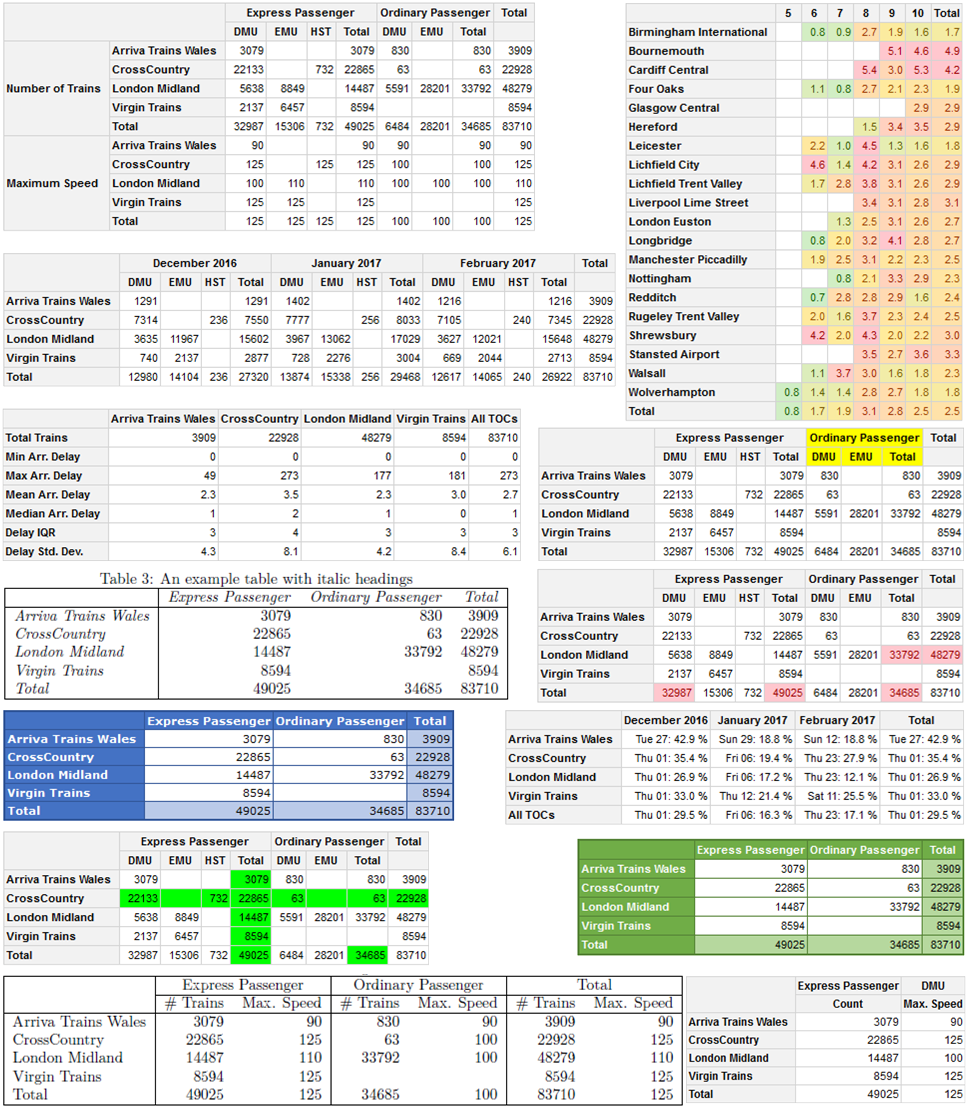The pivottabler package enables pivot tables to be created with just a few lines of R.

The pivottabler package aims to:

• Provide an easy way of creating pivot tables, without requiring the user to specify low-level layout logic.
• Provide multiple ways of specifying calculation logic to cover both simple and more sophisticated requirements.
• Provide styling options so the pivot tables can be themed/branded as needed.

All calculations for the pivot tables take place inside R, enabling the use of a wide-range of R functions in the calculation logic.

Pivot tables are rendered as htmlwidgets, Latex or plain text. The HTML/Latex/text can be exported for use outside of R.

Pivot tables can be converted to a standard R matrix or data frame. Pivot tables can be exported to Excel. Pivot tables can also be converted to a basictabler table for further manipulation.

pivottabler is a companion package to the basictabler package. pivottabler is focussed on generating pivot tables and can aggregate data. basictabler does not aggregate data but offers more control of table structure.

For more detailed information see http://www.pivottabler.org.uk/articles.

### Installation

You can install:

• the latest released version from CRAN with
install.packages("pivottabler")
• the latest development version from GitHub with
devtools::install_github("cbailiss/pivottabler", build_vignettes = TRUE)

### Example

pivottabler has many styling and formatting capabilities when rendering pivot tables in HTML / as htmlwidgets using pt$renderPivot(), however the most basic output is simply as plain text. #### Plain Text Output A simple example of creating a pivot table - summarising the types of trains run by different train companies: library(pivottabler) # arguments: qpvt(dataFrame, rows, columns, calculations, ...) qpvt(bhmtrains, "TOC", "TrainCategory", "n()") # TOC = Train Operating Company   Express Passenger Ordinary Passenger Total Arriva Trains Wales 3079 830 3909 CrossCountry 22865 63 22928 London Midland 14487 33792 48279 Virgin Trains 8594 8594 Total 49025 34685 83710  pivottabler also offers a more verbose syntax that is more self-describing and offers additional options that aren’t available with the quick-pivot functions. The equivalent verbose commands to output the same pivot table as above are: library(pivottabler) pt <- PivotTable$new()
pt$addData(bhmtrains) # bhmtrains is a data frame with columns TrainCategory, TOC, etc. pt$addColumnDataGroups("TrainCategory") # e.g. Express Passenger
pt$addRowDataGroups("TOC") # TOC = Train Operating Company e.g. Arriva Trains Wales pt$defineCalculation(calculationName="TotalTrains", summariseExpression="n()")
pt$evaluatePivot() pt Multiple levels can be added to the pivot table row or column headings, e.g. looking at combinations of TOC and PowerType: library(pivottabler) qpvt(bhmtrains, c("TOC", "PowerType"), "TrainCategory", "n()") library(pivottabler) pt <- PivotTable$new()
pt$addData(bhmtrains) pt$addColumnDataGroups("TrainCategory")
pt$addRowDataGroups("TOC") pt$addRowDataGroups("PowerType") # D/EMU = Diesel/Electric Multiple Unit, HST=High Speed Train
pt$defineCalculation(calculationName="TotalTrains", summariseExpression="n()") pt$evaluatePivot()
pt
                            Express Passenger  Ordinary Passenger  Total
Arriva Trains Wales  DMU                 3079                 830   3909
Total               3079                 830   3909
CrossCountry         DMU                22133                  63  22196
HST                  732                        732
Total              22865                  63  22928
London Midland       DMU                 5638                5591  11229
EMU                 8849               28201  37050
Total              14487               33792  48279
Virgin Trains        DMU                 2137                       2137
EMU                 6457                       6457
Total               8594                       8594
Total                                   49025               34685  83710  

#### HTML Output

The HTML rendering of the same two pivot tables shown above (each constructed using both a quick-pivot function and verbose syntax) is:

library(pivottabler)
qhpvt(bhmtrains, "TOC", "TrainCategory", "n()")
library(pivottabler)
pt <- PivotTable$new() pt$addData(bhmtrains)
pt$addColumnDataGroups("TrainCategory") pt$addRowDataGroups("TOC")
pt$defineCalculation(calculationName="TotalTrains", summariseExpression="n()") pt$renderPivot()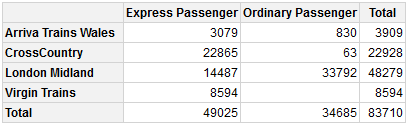library(pivottabler)
qhpvt(bhmtrains, c("TOC", "PowerType"), "TrainCategory", "n()")
library(pivottabler)
pt <- PivotTable$new() pt$addData(bhmtrains) # bhmtrains is a data frame with columns TrainCategory, TOC, etc.
pt$addColumnDataGroups("TrainCategory") # e.g. Express Passenger pt$addRowDataGroups("TOC") # TOC = Train Operating Company e.g. Arriva Trains Wales
pt$addRowDataGroups("PowerType") # D/EMU = Diesel/Electric Multiple Unit, HST=High Speed Train pt$defineCalculation(calculationName="TotalTrains", summariseExpression="n()")
pt$renderPivot()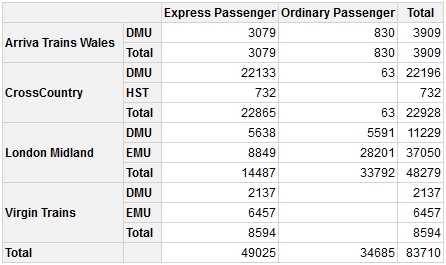Outline layout is an alternative way of rendering the row groups, e.g. for the same pivot table as above: library(pivottabler) pt <- PivotTable$new()
pt$addData(bhmtrains) pt$addColumnDataGroups("TrainCategory")
pt$addRowDataGroups("TOC", outlineBefore=list(isEmpty=FALSE, groupStyleDeclarations=list(color="blue")), outlineTotal=list(isEmpty=FALSE, groupStyleDeclarations=list(color="blue"))) pt$addRowDataGroups("PowerType", addTotal=FALSE)
pt$defineCalculation(calculationName="TotalTrains", summariseExpression="n()") pt$renderPivot()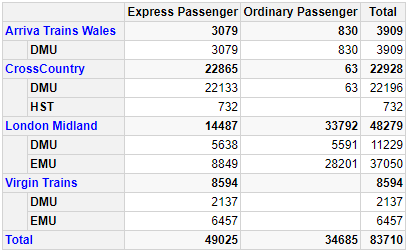Outline layout can also be used to build a pivot table with a variable depth hierarchy on the rows, e.g. a simple balance sheet: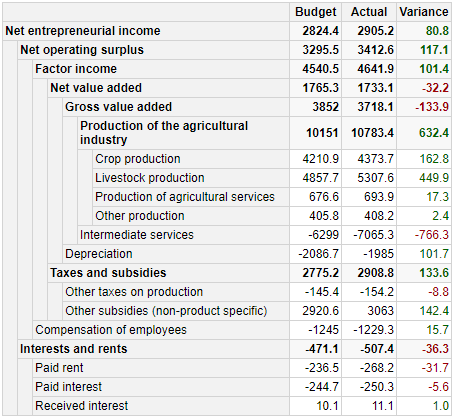The R for generating the above pivot table can be found in the Regular Layout vignette at http://www.pivottabler.org.uk/articles.

#### Multiple Calculations

Multiple calculations are supported. Calculations can be based on other calculations in the pivot table. Calculations can be hidden - e.g. to hide calculations that only exist to provide values to other calculations.

For example, looking at the total number of trains and the percentage of trains that arrive more than five minutes late for combinations of train operating company (TOC) and train category:

library(pivottabler)
library(dplyr)
library(lubridate)

# derive train delay data
trains <- mutate(bhmtrains,
ArrivalDelta=difftime(ActualArrival, GbttArrival, units="mins"),
ArrivalDelay=ifelse(ArrivalDelta<0, 0, ArrivalDelta),
DelayedByMoreThan5Minutes=ifelse(ArrivalDelay>=5,1,0))

# create the pivot table
pt <- PivotTable$new() pt$addData(trains)
pt$addRowDataGroups("TOC", totalCaption="All TOCs") pt$addColumnDataGroups("TrainCategory", totalCaption="All Trains")
pt$defineCalculation(calculationName="TotalTrains", caption="Train Count", summariseExpression="n()") pt$defineCalculation(calculationName="DelayedTrains", caption="Trains Arr. 5+ Mins Late",
summariseExpression="sum(DelayedByMoreThan5Minutes, na.rm=TRUE)",
visible=FALSE)
pt$defineCalculation(calculationName="DelayedPercent", caption="% Late Trains", type="calculation", basedOn=c("DelayedTrains", "TotalTrains"), format="%.1f %%", calculationExpression="values$DelayedTrains/values$TotalTrains*100") pt$renderPivot()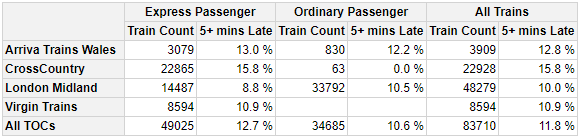It is also possible to change the axis (rows or columns) and level in which the calculations appear. See the “Calculations” vignette for details.

More advanced calculations such as % of row total, cumulative sums, etc are possible. See the “A2. Appendix: Calculations” vignette for details.

#### Styling Example

Styling can be specified when creating the pivot table. The example below shows specifying styling using a quick-pivot function and using the more verbose syntax.

library(pivottabler)
qhpvt(bhmtrains, "TOC", "TrainCategory", "n()",
tableStyle=list("border-color"="maroon"),
"font-style"="italic", "border-color"="maroon"),
cellStyle=list("color"="maroon", "background-color"="cornsilk",
"border-color"="maroon"),
totalStyle=list("color"="maroon", "background-color"="cornsilk",
"border-color"="maroon", "font-weight"="bold"))
library(pivottabler)
pt <- PivotTable$new(tableStyle=list("border-color"="maroon"), headingStyle=list("color"="cornsilk", "background-color"="maroon", "font-style"="italic", "border-color"="maroon"), cellStyle=list("color"="maroon", "background-color"="cornsilk", "border-color"="maroon"), totalStyle=list("color"="maroon", "background-color"="cornsilk", "border-color"="maroon", "font-weight"="bold")) pt$addData(bhmtrains)
pt$addColumnDataGroups("TrainCategory") pt$addRowDataGroups("TOC")
pt$defineCalculation(calculationName="TotalTrains", summariseExpression="n()") pt$renderPivot()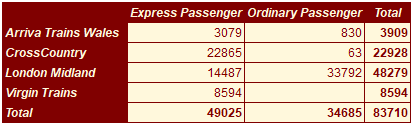It is also possible to change the styling of single cells and ranges of cells after the pivot table has been created. See the “Styling” and “Finding and Formatting” vignettes for more details.

#### Excel Output

The same styling/formatting used for the HTML output is also used when outputting to Excel - greatly reducing the amount of script that needs to be written to create Excel output.

library(pivottabler)
pt <- PivotTable$new() pt$addData(bhmtrains) # bhmtrains is a data frame with columns TrainCategory, TOC, etc.
pt$addColumnDataGroups("TrainCategory") # e.g. Express Passenger pt$addRowDataGroups("TOC") # TOC = Train Operating Company e.g. Arriva Trains Wales
pt$addRowDataGroups("PowerType") # D/EMU = Diesel/Electric Multiple Unit, HST=High Speed Train pt$defineCalculation(calculationName="TotalTrains", summariseExpression="n()")
pt$evaluatePivot() library(openxlsx) wb <- createWorkbook(creator = Sys.getenv("USERNAME")) addWorksheet(wb, "Data") pt$writeToExcelWorksheet(wb=wb, wsName="Data",
topRowNumber=2, leftMostColumnNumber=2, applyStyles=TRUE)
saveWorkbook(wb, file="C:\\test.xlsx", overwrite = TRUE)In the screenshot above, Gridlines have been made invisible to make the styling easier to see (by clearing the checkbox on the ‘View’ ribbon). Columns were also auto-sized - though the widths of columns could also be manually specified from R. See the Excel Export vignette for more details.